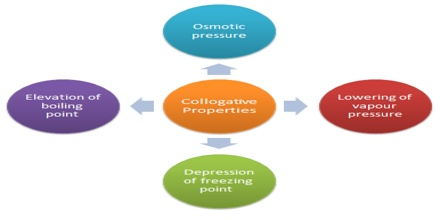# Colligative Properties

Colligative Properties

If the concentration of the solute in a solution is very low, the solution is called a dilute solution. Four properties of dilute solutions are so intimately connected with each other that they are grouped together and are commonly known as the ‘colligative properties’ (colligative means “tied together”). These four properties are:

(ii) Elevation of boiling point,

(iii) Depression of freezing point and

(iv) Osmotic pressure.All the four properties depend only on the number of particles (molecules or ions) in a solution, but they are independent of molecular structure. A litre of solution containing one mole of sodium chloride contains twice the number of particles if one mole of sugar molecules dissolved in the same volume of solution. This is because each sodium chloride unit gives two particles, one Na+ ion and one Cl ion, and the abase solution will contain two moles of particles. The property of the sodium chloride solution will, therefore, be twice the value of the same property of a solution of sugar of the same concentration. A solution of urea (RMM = 60) has the same value of the properties as that of a solution of sucrose (RMM = 342) of the same concentration in mol L-1. Pressure exerted by a gas is also independent of molecular structure and so the gas pressure or volume should be termed as colligative properties. However, colligative properties have almost universally been associated with dilute solutions.

The properties are quantitatively expressed in the form of certain laws, the validity of which is restricted to dilute solutions. One use of the study of these properties is that the molecular mass of solutes may be determined from measurements of any one of them with the help of these laws.